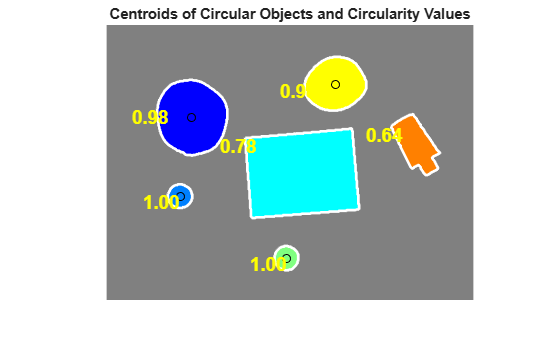# Identifying Round Objects

This example shows how to classify objects based on their roundness using `bwboundaries`, a boundary tracing routine.

### Step 1: Read an Image

Read in `pills_etc.png`.

```RGB = imread("pillsetc.png"); imshow(RGB)```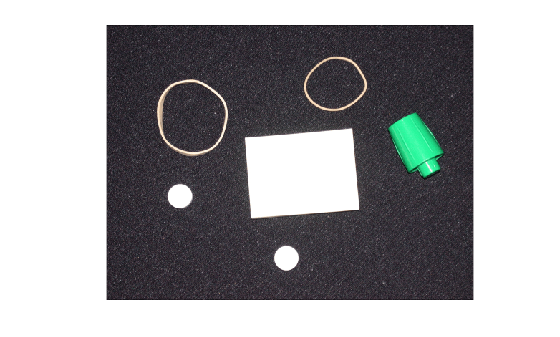### Step 2: Threshold the Image

Convert the image to black and white in order to prepare for boundary tracing using `bwboundaries`.

```I = im2gray(RGB); bw = imbinarize(I); imshow(bw)```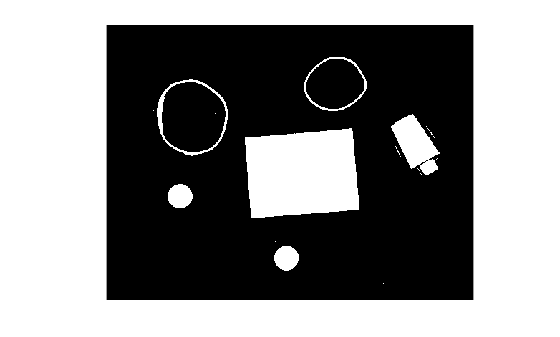### Step 3: Preprocess the Image

Using morphology functions, remove pixels which do not belong to the objects of interest.

Remove all objects containing fewer than 30 pixels.

```minSize = 30; bw = bwareaopen(bw,minSize); imshow(bw)```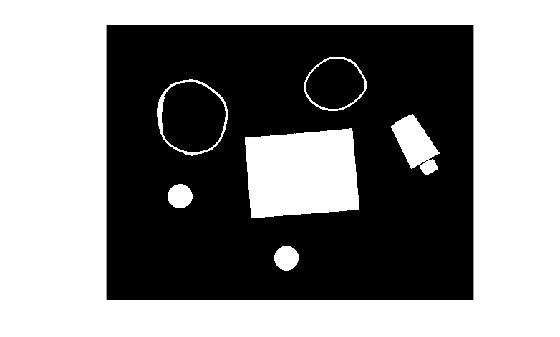Fill a gap in the pen's cap.

```se = strel("disk",2); bw = imclose(bw,se); imshow(bw)```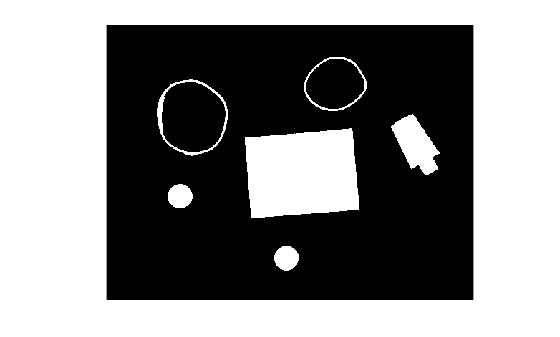Fill any holes, so that `regionprops` can be used to estimate the area enclosed by each of the boundaries

```bw = imfill(bw,"holes"); imshow(bw)```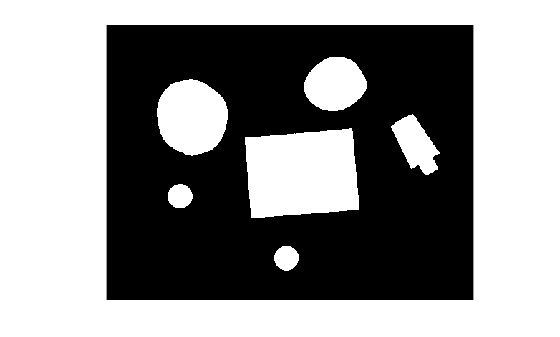### Step 4: Find the Boundaries

Concentrate only on the exterior boundaries. Specifying the "`noholes"` option will accelerate the processing by preventing `bwboundaries` from searching for inner contours.

`[B,L] = bwboundaries(bw,"noholes");`

Display the label matrix and draw each boundary.

```imshow(label2rgb(L,@jet,[.5 .5 .5])) hold on for k = 1:length(B) boundary = B{k}; plot(boundary(:,2),boundary(:,1),"w",LineWidth=2) end title("Objects with Boundaries in White")```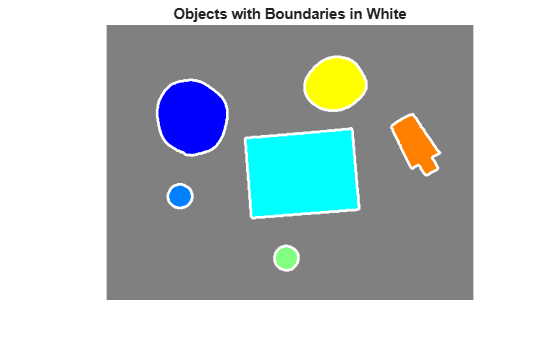### Step 5: Determine which Objects are Round

Estimate the circularity and centroid of all of the objects using the `regionprops` function. The circularity metric is equal to 1 for an ideal circle and it is less than 1 for other shapes.

`stats = regionprops(L,"Circularity","Centroid");`

The classification process can be controlled by setting an appropriate threshold. In this example, use a threshold of `0.94` so that only the pills will be classified as round.

`threshold = 0.94;`

Loop over the detected boundaries. For each object:

• Obtain the (x,y) boundary coordinates and the circularity measurement

• Compare the circularity measurement to the threshold. If the circularity exceeds the threshold, calculate the position of the centroid and display the centroid as a black circle.

• Display the circularity measurement in yellow text over the object.

```for k = 1:length(B) % Obtain (X,Y) boundary coordinates corresponding to label "k" boundary = B{k}; % Obtain the circularity corresponding to label "k" circ_value = stats(k).Circularity; % Display the results circ_string = sprintf("%2.2f",circ_value); % Mark objects above the threshold with a black circle if circ_value > threshold centroid = stats(k).Centroid; plot(centroid(1),centroid(2),"ko"); end text(boundary(1,2)-35,boundary(1,1)+13,circ_string,Color="y",... FontSize=14,FontWeight="bold") end title("Centroids of Circular Objects and Circularity Values")```